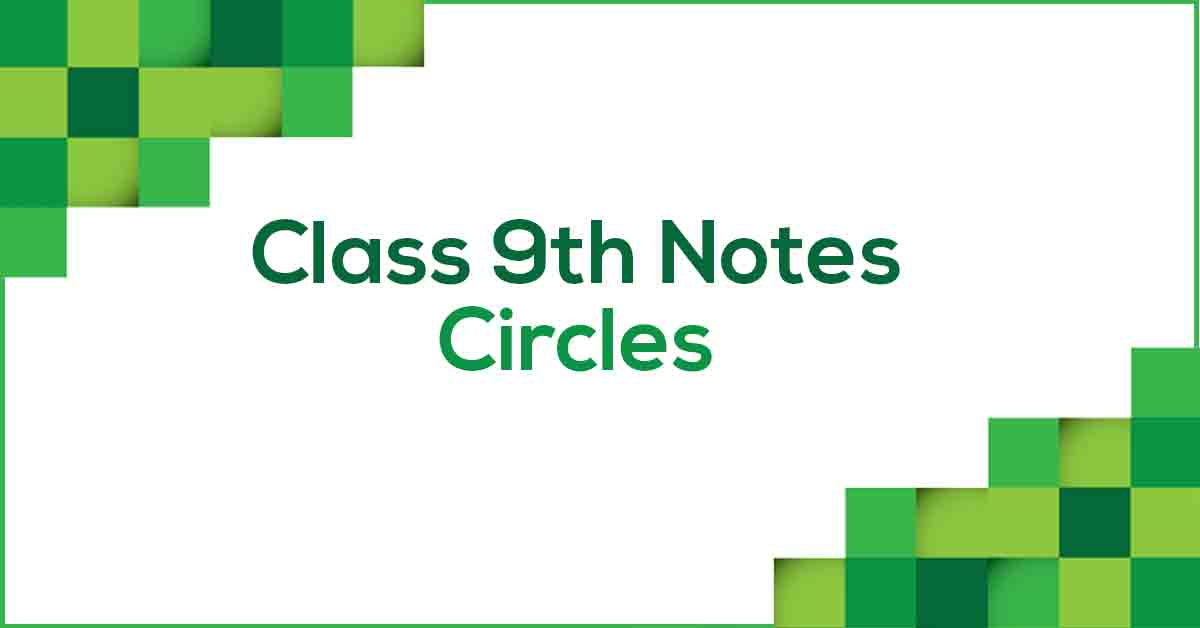Circles Class 9 NotesClass 9 Maths Chapter 10 Circles Notes - PDF Download

Circles Class 9 Notes are prepared according to the latest NCERT Syllabus and provide the students with the summary of the chapter, important points to remember and detailed explanation of important concepts and derivations for better understanding and retaining of the chapter. Circles Class 9 Notes would ease out your learning and revision process.

How do you define Circles?

A circle is a simple closed shape. It is the set of all points in a plane that are at a given distance from a given point, the centre; equivalently it is the curve traced out by a point that moves so that its distance from a given point is constant. In this chapter, we will learn about all the theorems and properties of circles.

Learn more about the same in Circles Class 9 Notes pdf.